# 插件分享 | 一键统计 Windows 各版本数量的 Windows Count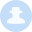GobySec  48天前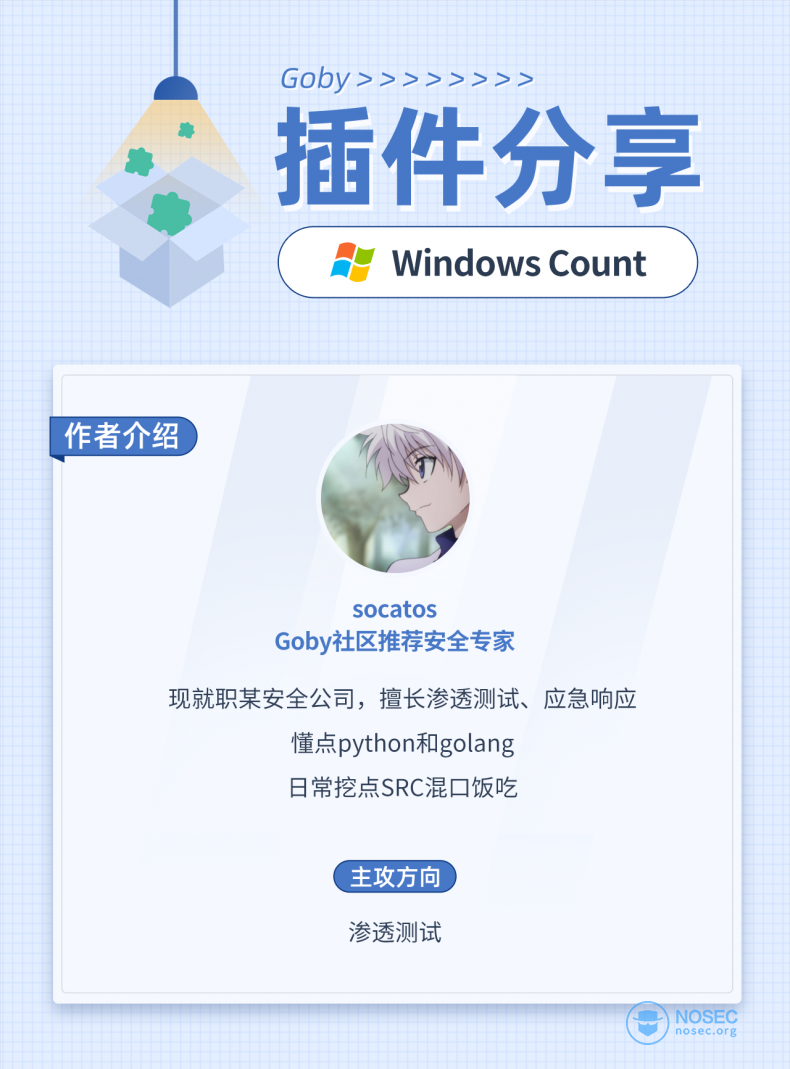Goby社区第16 篇插件分享文章

# 0×01 插件使用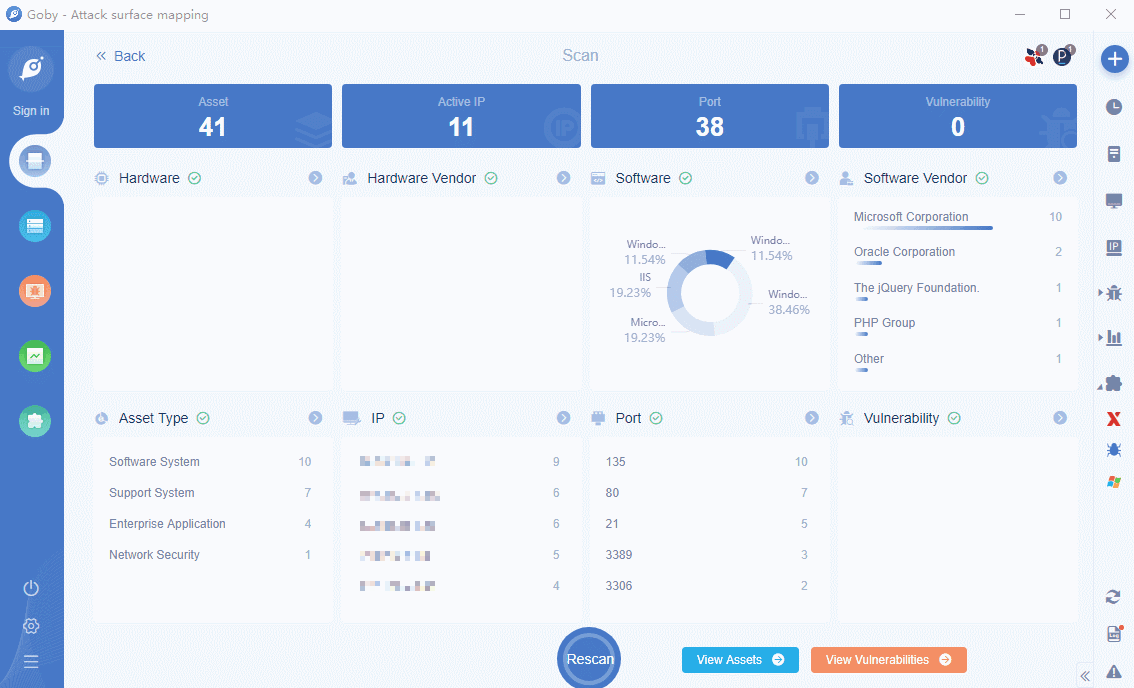# 0×02 插件开发

## 2.1 开发思路

• 插件基本配置

• 从扫描资产中提取信息

• Windows 版本信息提取

• 提取 IP 和数量

• 展示

## 2.2 插件基本配置

``````function activate (content) {
goby.registerCommand('WindowsCount', function () {#WindowsCount以带要和package中的command值对应
let path = __dirname + "/os.html"#实现的代码文件路径
goby.showPage(path,false);#展示以页面方式展示
});
}

exports.activate = activate;``````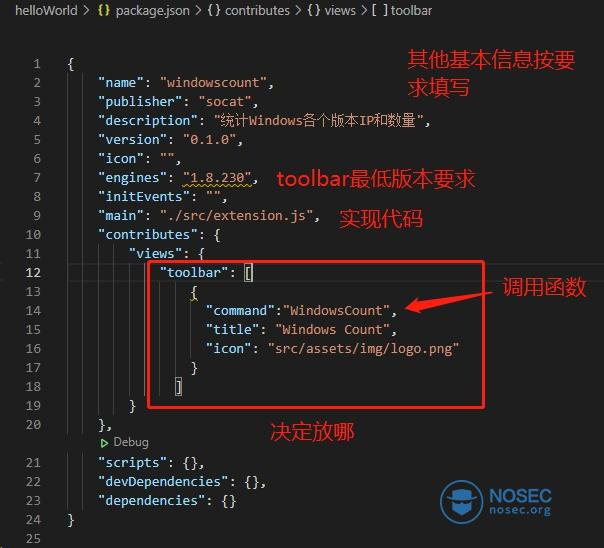## 2.3 从扫描资产中提取信息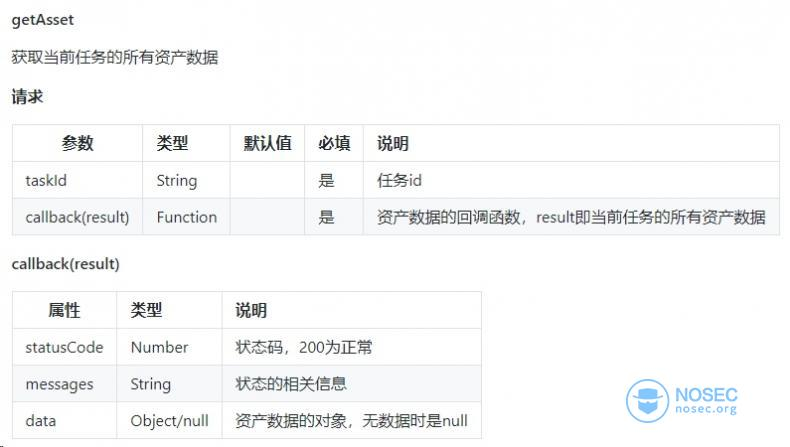``````goby.getAsset(goby.getTaskId(), getAssetData);//获取资产数据
function getAssetData(data) {
if (data.statusCode == 200) {
console.log(data);//打印出返回的data数据
}
}``````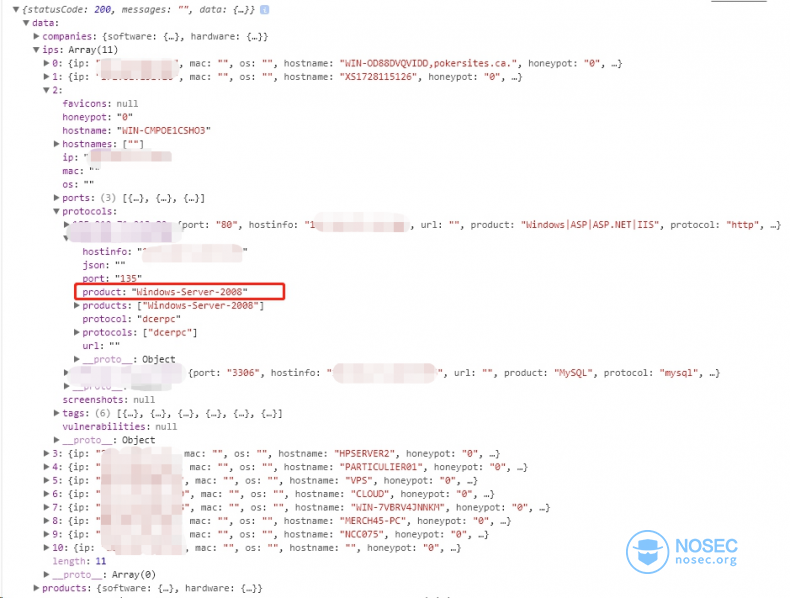## 2.4 Windows 版本信息提取

``````let ostypes = []; //存储系统类型
//去重函数
function unique(arr) {
return Array.from(new Set(arr));
}
function getAssetData(data) {
if (data.statusCode == 200) {
let ipinfo = data.data.ips
//获取OS类型
ipinfo.forEach((v, k) = >{
if (v.protocols) {
for (var i in v.protocols) {
if (v.protocols[i].protocol.search("dcerpc") != -1 && v.protocols[i].product.search("Windows") != -1) {
console.log(v.protocols[i].product)
ostypes.push(v.protocols[i].product);//将系统类型存入列表中
}
}
}
});
ostypes = unique(ostypes);//去重
}
}``````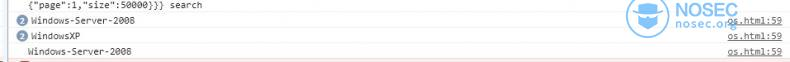## 2.5 提取 IP 和数量

``````        //根据OS类型生成dic
for (var i in ostypes) {
osinfo[ostypes[i]] = [];
}

//根据OS类型匹配对应的IP
for (var key in osinfo) {
ipinfo.forEach((v, k) => {
if (v.protocols) {
for (var i in v.protocols) {
if (v.protocols[i].port == 135 && v.protocols[i].product == key) {
osinfo[key].push(v.protocols[i].hostinfo.split(":"));
}
}
}
});

}
console.log(osinfo)``````## 2.6 展示

``````<!DOCTYPE html>
<html lang="en">

<meta charset="UTF-8">
<meta name="viewport" content="width=device-width, initial-scale=1.0">
<title>Windows系统统计表</title>

<body>
<div>
<script>
</script>
</div>
<div>
<table id="os-table" class="layui-table" lay-even lay-skin="nob">
<tr>
<th width="40" style="font-size: medium;" data-i18n="System type"></th>
<th width="55" style="font-size: medium;">IP</th>
<th width="55" style="font-size: medium;" data-i18n="Count"></th>
</tr>
<tbody>
<tr>
</tr>
</tbody>
</table>
</div>
</div>

<script src="assets/js/jquery-3.3.1.min.js"></script>
<script src="assets/lib/layui/layui.all.js"></script>
<script src="assets/js/jquery.i18next.js"></script>
<script>
let osinfo = new Array();
let fs = parent.require('fs');
let ostypes = [];
let html = "";

//去重函数
function unique(arr) {
return Array.from(new Set(arr));
}

function lang(){
//获取当前Languzge
let language = goby.getLang();

//判断翻译文件是否存在
let translateState = fs.existsSync(goby.__dirname + '/assets/translate/' + language+'/html.json');

//翻译文件存在则使用翻译文件,否则使用默认EN翻译
let lang = translateState?language:'EN';

let a = \$.i18n.init({
lng: language, //指定语言
resGetPath: './assets/translate/'+lang+'/html.json',//语言包的路径
}, function(err, t) {
if(!err){
\$('[data-i18n]').i18n(); // 通过选择器集体翻译
return;
}
goby.showErrorMessage(err)
});
}

lang();

//当lang改变时更新页面内容
goby.bindEvent('on changeLang',()=>{
lang();
})

function getAssetData(data) {
if (data.statusCode == 200) {
let ipinfo = data.data.ips

//获取OS类型
ipinfo.forEach((v, k) => {
if (v.protocols) {
for (var i in v.protocols) {
if (v.protocols[i].protocol.search("dcerpc") != -1 && v.protocols[i].product.search("Windows") != -1) {
ostypes.push(v.protocols[i].product);
}
}
}
});
ostypes = unique(ostypes);

//根据OS类型生成dic
for (var i in ostypes) {
osinfo[ostypes[i]] = [];
}

//根据OS类型匹配对应的IP
for (var key in osinfo) {
ipinfo.forEach((v, k) => {
if (v.protocols) {
for (var i in v.protocols) {
if (v.protocols[i].protocol.search("dcerpc") != -1 && v.protocols[i].product == key) {
osinfo[key].push(v.protocols[i].hostinfo.split(":"));
}
}
}
});

}

//输出数据

for (var os in osinfo) {
let ips = osinfo[os];
let nums = osinfo[os].length;
let tds = "";
for (var ip in ips) {
tds += ips[ip] + "</br>";
}

html += `<tr>
<td>\${os}</td>
<td>\${tds}</td>
<td>\${nums}</td>
</tr>`;
}
\$("#os-table tbody").html(html);

}
}
</script>
</body>

</html>``````# 0×03 小结

https://gobies.org/docs.html

https://github.com/gobysec/GobyExtension/projects

#### 最新评论